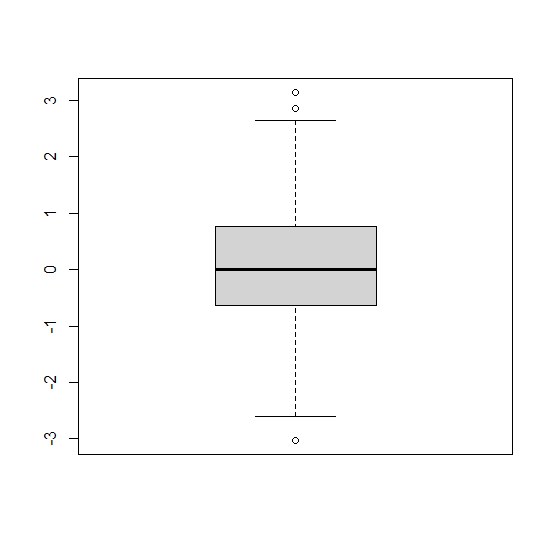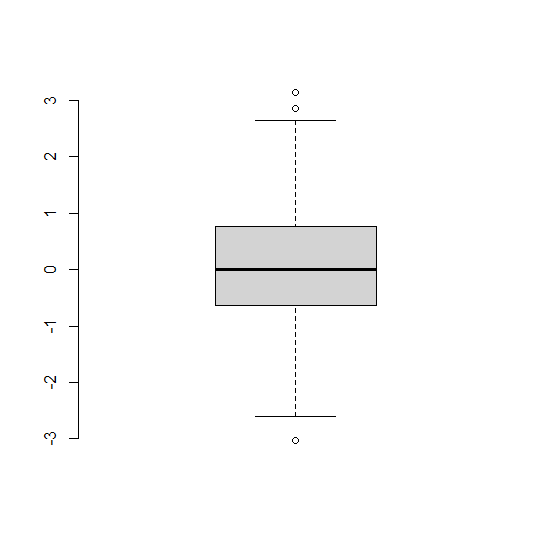# How to create a boxplot without frame in base R?

The boxplot in base R is covered with a box and that box is called a frame. We can get rid of that frame by using frame argument while creating the boxplot. For example, if we have a vector called x and we want to create the boxplot without frame then it can be done by using the command boxplot(x,frame=F). This will remove all the sides of the boxplot except the Y−axis labels because this will help us to understand the distribution of the variable.

## Example

Live Demo

x<−rnorm(500)
boxplot(x)

## OutputCreating the boxplot without frame −

## Example

boxplot(x,frame=F)

## Output